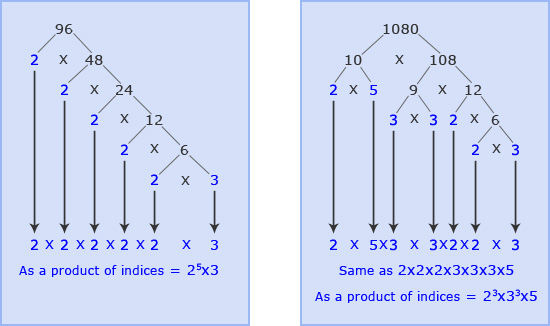## Prime Factorisation

Prime Factorisation is also known as Prime Factor Decomposition, or Writing as a Product of Primes)

Prime Factorisation is when we find the factors of numbers, but we only use prime numbers when we pair up the factors. At the end, you must be able to multiply all the prime factors to give you your original number – this is a useful tool for checking you haven’t made any silly mistakes.

Finding the prime factorisation of 96 can be set out as below: always start with 2 (as it always easy to halve a number) and highlight your prime numbers as you go along, so you know not to carry on with that branch.

Similarly, the prime factors for 1080 can be set out as below. In this case where the number is big it is easier to break it down in the way shown below, but remember to only stop along a branch when both numbers are prime.

To find the HCF and LCM of 2 numbers, finding the prime factors first is the easiest way. If we continue with the example of 96 and 1080 if we list the prime factors calculate above we get:When considering the HCF and LCM, using a Venn diagram can make it easier to see which factors are shared: